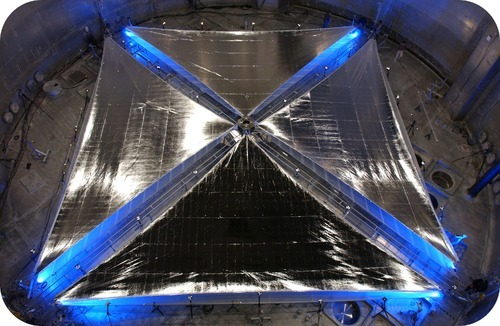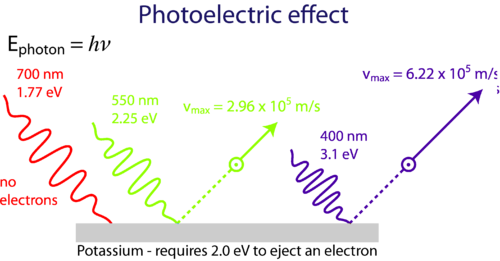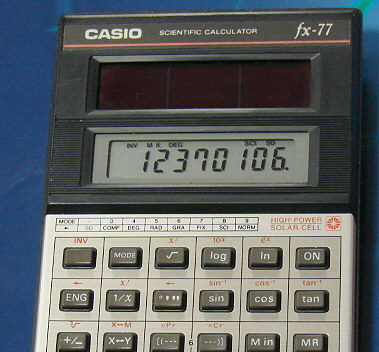## Photoelectric Effect

### Learning Objectives

• Describe the photoelectric effect.

### Are you a fan of science fiction?

In old science fiction stories (1950s), one of the space travel themes was the use of solar sails for propulsion. The idea was that the photon pressure from the sun would push the sail (like the wind sails) and move the space craft. What once was science fiction is now reality as solar sails are being developed and tested for modern space travel.## Photoelectric Effect and the Particle Nature of Light

In 1905 Albert Einstein (1879–1955) proposed that light be described as quanta of energy that behave as particles. A photon is a particle of electromagnetic radiation that has zero mass and carries a quantum of energy. The energy of photons of light is quantized according to the Ehv equation. For many years light had been described using only wave concepts, and scientists trained in classical physics found this wave-particle duality of light to be a difficult idea to accept. A key experiment that was explained by Einstein using light’s particle nature was called the photoelectric effect.

The photoelectric effect is a phenomenon that occurs when light shined onto a metal surface causes the ejection of electrons from that metal. It was observed that only certain frequencies of light are able to cause the ejection of electrons. If the frequency of the incident light is too low (red light, for example), then no electrons were ejected even if the intensity of the light was very high or it was shone onto the surface for a long time. If the frequency of the light was higher (green light, for example), then electrons were able to be ejected from the metal surface even if the intensity of the light was very low or it was shone for only a short time. This minimum frequency needed to cause electron ejection is referred to as the threshold frequency.

Classical physics was unable to explain the photoelectric effect. If classical physics applied to this situation, the electron in the metal could eventually collect enough energy to be ejected from the surface even if the incoming light was of low frequency. Einstein used the particle theory of light to explain the photoelectric effect as shown in the Figure below.Figure 1. Low frequency light (red) is unable to cause ejection of electrons from the metal surface. At or above the threshold frequency (green) electrons are ejected. Even higher frequency incoming light (blue) causes ejection of the same number of electrons but with greater speed.Figure 2. Photoelectric cells convert light energy into electrical energy which powers this calculator.

Consider the Ehv equation. The E is the minimum energy that is required in order for the metal’s electron to be ejected. If the incoming light’s frequency, v, is below the threshold frequency, there will never be enough energy to cause electron to be ejected. If the frequency is equal to or higher than the threshold frequency, electrons will be ejected.

As the frequency increases beyond the threshold, the ejected electrons simply move faster. An increase in the intensity of incoming light that is above the threshold frequency causes the number of electrons that are ejected to increase, but they do not travel any faster. The photoelectric effect is applied in devices called photoelectric cells, which are commonly found in everyday items such as a calculator which uses the energy of light to generate electricity.

### Summary

• Light has properties of both a wave and a particle.
• The photoelectric effect is produced by light striking a metal and dislodging electrons form the surface of the metal.

### Practice

Use the link below to answer the following questions:

http://www.citycollegiate.com/physicsXII_photocell.htm

1. What is the emitting material in this photocell?
2. What causes photoelectrons to be emitted?
3. Does the current increase or decrease with an increase in light intensity?
4. What happens if light does not strike the cathode?

### Review

1. What are the properties of a photon?
2. What does the photoelectric effect show about the properties of light?
3. How does the frequency of light affect the release of photons?

## Glossary

• photoelectric cell: Use energy of light to generate electricity.
• photoelectric effect: A phenomenon that occurs when light shined onto a metal surface causes the ejection of electrons from that metal.
• photon: A particle of electromagnetic radiation that has zero mass and carries a quantum of energy.
• threshold frequency: Minimum frequency needed to cause electron ejection.